71. The complexity for: 𝑇(𝑛 ) = 𝑇(𝑛 −1)+𝑛 , is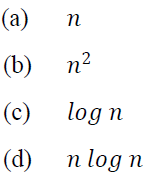72. Which one of the following algorithmic approaches is followed in Floyd-Warshall shortest path algorithm?
(a) Divide and conquer
(b) Dynamic programming
(c) Greedy approach
(d) Backtracking

73. The worst-case complexity to sort an array of integers in non-decreasing order, by using quicksort is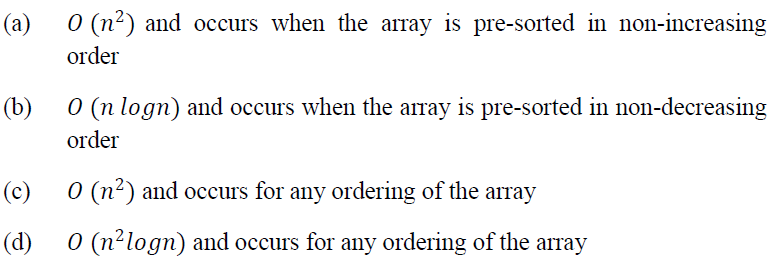74. What allows the Java programmer to destroy an object A?
(a) a. delete ( )
(b) a. finalize ( )
(c) Runtime. GetRuntime ( ). gc ( )
(d) Only the garbage collection system can destroy an object

75. In which order a Binary search tree should be traversed to obtain the output sequence in descending order?
(a) Root, left and right
(b) Right, root and left
(c) Right, left and root
(d) Left, root and right

76. The total number of comparisons made in Bubble sort algorithm is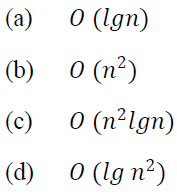77. The recurrence relation for the optimal execution time of the Tower of Hanoi problem having n discs is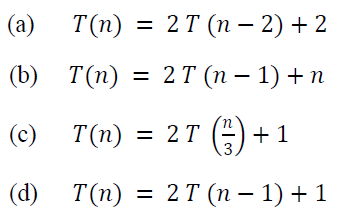78. The running time of an algorithm 𝑇(𝑛 ) where 𝑛 the input size is given by: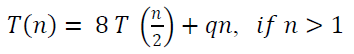= 𝑝 , if 𝑛 = 1
where, 𝑝 and 𝑞 are constants.

What is the complexity (order) of the algorithm?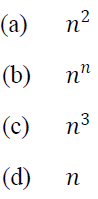79. Consider the following statements regarding automata theory:
1. The pumping length must always be equal to the number of states in a machine
2. A non-regular expression can have a finite pumping length
3. In a regular language/expression, a string of pumping length can be repeated arbitrarily
4. The language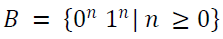has a pumping length

Which of the above statements is/are correct?
(a) 1, 2 and 4
(b) 1, 3 and 4
(c) 2 only
(d) 3 only

80. Consider the following machines regarding Finite automatas:
1. DFA
2. NFA
3. E - NFA
4. Any automaton

Which of the above are correct about the applicability of Arden’s Theorem?
(a) 1 and 4
(b) 1 and 2
(c) 2 and 3
(d) 3 and 4